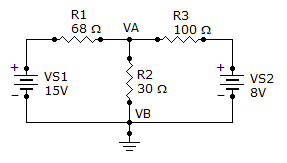# Electrical Engineering - Branch, Loop and Node Analyses - Discussion

Discussion Forum : Branch, Loop and Node Analyses - General Questions (Q.No. 2)
2.
What is the current through R2?3.19 A
319 mA
1.73 A
173 mA
Explanation:
No answer description is available. Let's discuss.
Discussion:
16 comments Page 1 of 2.

Pente vinodkumar said:   8 years ago
By applying loop analysis at first loop.

15 = 68i'+ 30(i'+i").
15 = 98i'+ 30i".

Now at second loop:

8 = 100i"+30*(i'+i").
8 = 30i'+130i".

After calculation:

i' = 0.145.
i" = 0.028.

So total current in 30 ohm resistance.

i' + i".

0.145 + 0.028.

= 173 mA.
(2)

Ashish singhal said:   1 decade ago
By appling mesh analysis

15=68i'+ 30(i'-i")
15=98i'-30i"

Now at second battery

-8=100i"+30*(i'-i")
-8=-30i'+130i"

After calculation

i'=.145
i"= -.028

So total current in 30 ohm resistance

i' - i"

0.145 - (-0.028)

= 0.173 A or

= 173 mA.

Somashekhar y said:   8 years ago
((va-12)/49)+(va/24)+((va-6)/80) = 0.
((va-12)(1920)+(va)(3920)+(va-6)(1176))/94080 = 0.

va(7016)-30096 = 0.
va = 30096/7016.
va = 4.28.

Please review previous question. Why nodal equation differs? Can any one explain?

Sunilkumar v said:   1 decade ago
Apply nodal analysis for the circuit. After getting Va value, we can find current at 30 ohm.res

(15-VA)/68 - (VA-0)/30 - (VA-8)/100 = 0.

VA = 5.169.

R2 = VA/30.

R2 = 5.169/30.

R2 = 173 mA.
(2)

Akinremi Akinola Musbau said:   8 years ago
@Shinghal.

Your working is right but take note that the current through 30 ohms is the sum of the current that emanate from the two cell and not the difference.

Applying kvl to the circuit,

((v-15)/68)+(v/30)+((v-8)/100).
On solving above expression we get 5.171v.

Current at R2= 5.17/30=0.172 amps.
172 milli amps.

Rita J said:   1 decade ago
Applying kcl at node A and taking node B as refrence:

(15-va)/68-va/30-(va-8)/100=0

va=5.1689v;

Ir2=va/30=5.1689/30=0.172A=172mA;

Dinesh kumar t said:   1 decade ago
Applying kcl at node A and taking node B as refrence:

(15-va)/68-va/30-(va-8)/100=0

va=5.1689v;

Ir2=va/30=5.1689/30=0.172A=172mA.

Krishna said:   8 years ago
The answer must me same irrespective of method we acquire but I did't get answer using mesh analysis.

Julius said:   9 years ago
Can you please elaborate the solution so that I can understand. Thanks.